Detectors such as rotary encoder, liner encoder, flow meter output variation in pulse signal. It is converted into physical values on the measuring equipment side and displayed numerically.
We introduce Event counter function and F/V converter function which enable to convert pulse signal into physical value in this column.
F/V converter of OS-2000 series is specialized to read rotational pulse data, but also enables to covert frequency of other pulse signal into distance, speed and flow.
Here we take pulse signal from liner encoder as example to obtain moving distance (event counter) and instantaneous speed (F/V converter).

If it is a flow meter, it corresponds to total flow rate/ instantaneous flow rate. This function has wide application range.

Pulse signal is 0.5mm/pulse.
If 100 pulse outputs, the moving distance is 100 x 0.5mm= 50mm.

This is signal wave. The moving speed is getting fast and after a certain speed, it slows down.
(The higher the recording sample frequency, the higher the resolution of the F / V converter.)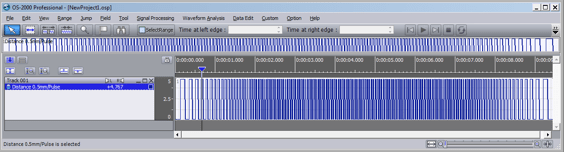Pulse wave

1.The method to check moving distance (total value) against time with Event Counter

Select [Event Counter] from Signal Processing Menu.
Set Initial count value 1, Counter step value 1.
Select condition file and click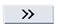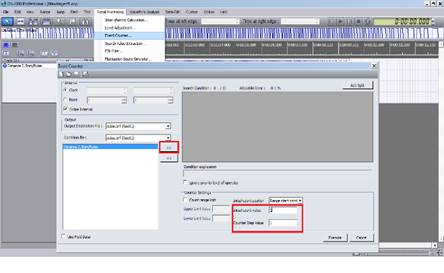In Search condition setting dialog box, set as follow;
Condition type: Level Trigger
Trigger level: 50% of pulse waveform amplitude
Hysteresis: smaller value than trigger level (ignore minor changes)
Click [OK] to return to [Event Counter] dialog box, then click [Execute].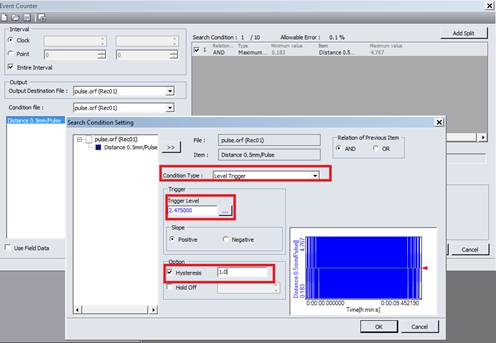A new data is created and displayed in Data Manager.
Pulse data is shown as count value yet.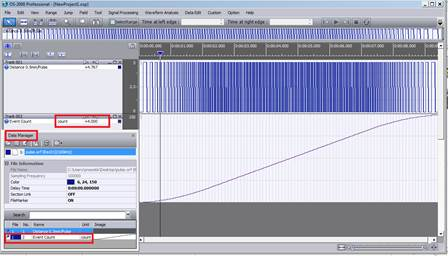As mentioned, the moving distance is 0.5 mm/pulse. Thus, the count value times 0.5 is moving distance.

Use [Simple calculation].
Set , 0.5 in calculation expression setting, tick convert unit box and set mm.
Then click [Execute].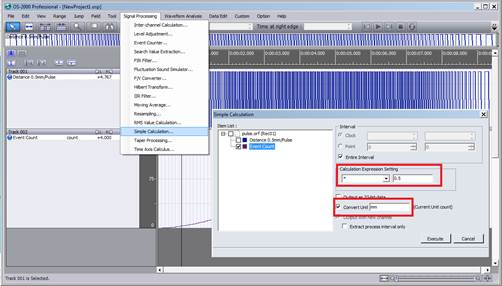Pulse data is converted to moving distance value.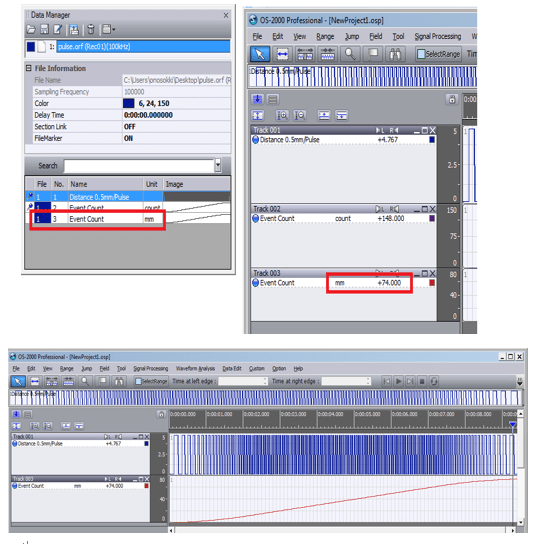Reading cursor, it finds moving distance 74 mm.

2. The method to check instantaneous speed with F/V converter

Select [F/V converter] from Signal Processing Menu.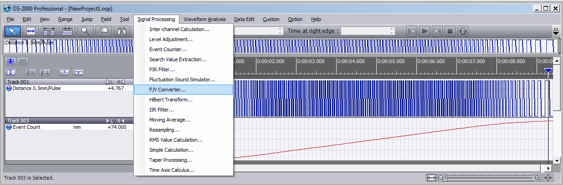F/V converter is designed to convert rotational speed but also other signals.
Since the rotation speed unit is r/min, it is calculated as a value per minute.

Set
Conversion type: Pulse
Pulse/Revolution: Normally the number of pulses per rotation but 120= 2 x 60
(2 pulse/mm x 60 sec = distance/minute this time)
Level: 50% of pulse waveform
Hysteresis: smaller value than trigger level (ignore minor changes)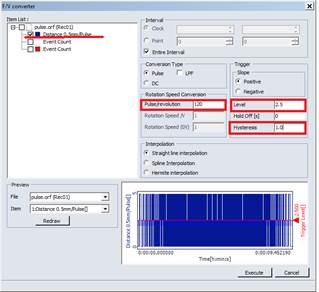After click [Execute], a new data is created as below.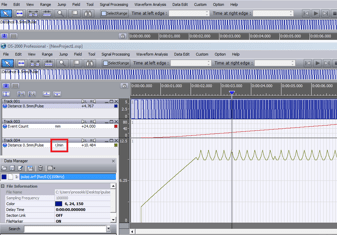In the section where the pulse interval is changing, you can read how the speed changes while moving.
The unit is r/min yet, thus change to mm/s as follow;
Select [Channel setting] from Data Edit Menu.
Key in mm/s into Unit to change signal type to Speed.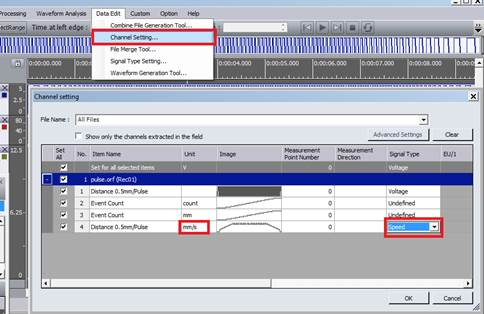Now distance value (mm/s) is displayed.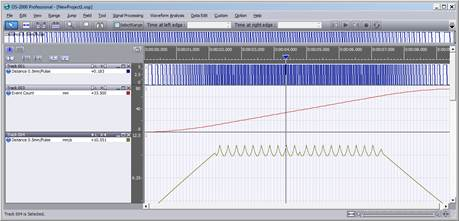In this way, the physical value of the pulse signal can be easily obtained by the conversion function of OS-2000 series. Fluctuating frequency can also be obtained by performing frequency analysis. Physical values changing cannot be viewed in real-time, but if pulse signals are recorded, various secondary processing analysis can be performed later. Total flow rate and instantaneous flow rate are obtained by pulse signal of flow meter.

If pulse signals from rotation, distance, flow rate and torque can be recorded, OS-2000 series can be used in many situations.

(H.K)